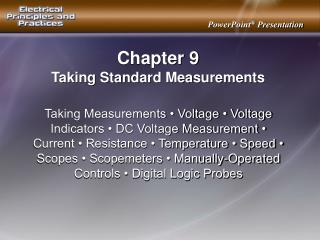DownloadDownload PresentationChapter 9

# Chapter 9

Télécharger la présentation## Chapter 9

- - - - - - - - - - - - - - - - - - - - - - - - - - - E N D - - - - - - - - - - - - - - - - - - - - - - - - - - -
##### Presentation Transcript

1. Chapter 9 Taking Standard Measurements Taking Measurements • Voltage • Voltage Indicators • DC Voltage Measurement • Current • Resistance • Temperature • Speed • Scopes • Scopemeters • Manually‑Operated Controls • Digital Logic Probes

2. A test light is a test instrument with a bulb that is connected to two test leads to give a visual indication when voltage is present in a circuit.

3. When testing a receptacle, the test light bulb illuminates when the receptacle is properly wired and energized.

4. A receptacle tester is a device that is plugged into a standard receptacle to determine if the receptacle is properly wired and energized.

5. Refer to the operating manual of the test instrument for all measuring precautions limitations, and procedures.

6. A voltage indicator is a test instrument that indicates the presence of voltage when the test tip touches, or is near, an energized hot conductor or energized metal part.

7. Always follow a voltage indicator test with a test using a voltmeter to verify the voltage indicator measurement any time the voltage indicator indicates no voltage.

8. A voltage tester is an electrical test instrument that indicates the approximate voltage amount and type of voltage (AC or DC) in a circuit by the movement of a pointer (and vibration on some models).

9. Before taking any voltage measurements using a voltage tester, ensure the tester is designed to take measurements on the circuit being tested.

10. Exercise caution when measuring AC voltages over 24 V.

11. Exercise caution when measuring DC voltages over 60 V.

12. Care must be taken to protect the meter, circuit, and person using the meter when measuring DC with an in‑line ammeter.

13. Clamp‑on ammeters measure the current in a circuit by measuring the strength of the magnetic field around a conductor.

14. A megohmmeter detects insulation failure or potential failure of insulation caused by excessive moisture, dirt, heat, cold, corrosive substances, vibration, and aging.

15. The amount of temperature at a location can be measured using a contact thermometer or infrared meter.

16. A contact thermometer is an instrument that measures temperature at a single point.

17. An infrared meter is a meter that measures heat energy by measuring the infrared energy that a material emits.

18. The speed of a rotating object can be measured using a contact tachometer, photo tachometer, or strobe tachometer.

19. A contact tachometer is a device that measures the rotational speed of an object through direct contact of the tachometer tip with the object to be measured

20. A photo tachometer is a device that measures the speed of an object without direct contact with the object.

21. A strobe tachometer is a device that uses a flashing light to measure the speed of a moving object.

22. The two basic types of scopes used in troubleshooting are oscilloscopes and scopemeters.

23. A trace is a reference point/line that is visually displayed on the face of the scope screen.

24. Typical manually‑operated scope adjustment controls include intensity, focus, horizontal positioning, vertical positioning, volts/division, and time/division.

25. The volts/division (volts per division) control selects the height of the displayed waveform.

26. The time/division (time per division) control selects the width of the displayed waveform.

27. A scope is connected by a probe on the end of a test lead. A 1X probe (1 to 1) is used to connect the input of the scope to the circuit under test when the test voltage is lower than the voltage limit of the scope.

28. A scopemeter gives an adequate reading in most frequency measurement applications.

29. To measure AC voltage using a scope, a test probe is connected to the point in the circuit where the DC voltage is to be measured.

30. A digital logic probe is a special DC voltmeter that detects the presence or absence of a signal.

31. A power supply voltage is checked with voltmeter if the digital circuit or logic probe has intermittent problems.